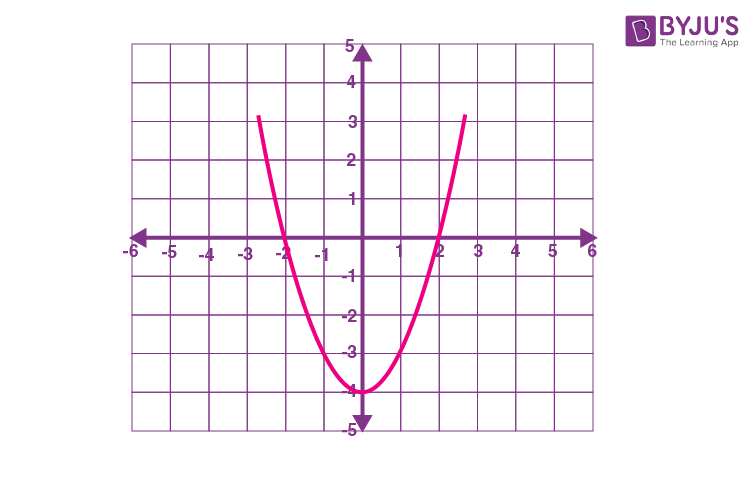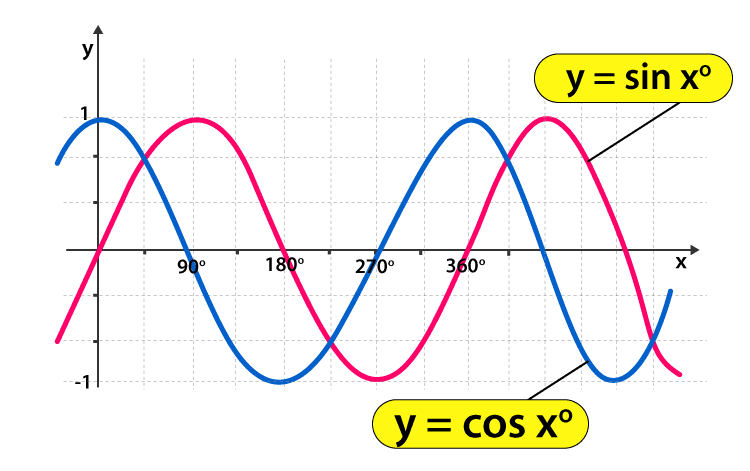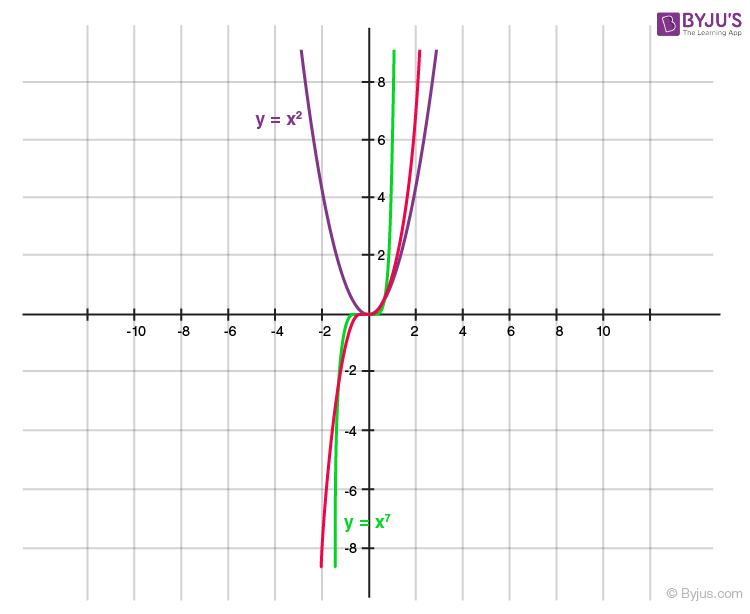Defend yourself better by mastering the science of immunity and vaccines. Secure your free spot, now! Defend yourself better by mastering the science of immunity and vaccines. Secure your free spot, now!

# Even Function

A function can be defined as even, odd or neither in different ways, either algebraically or graphically. A function is called an even function if its graph is unchanged under reflection in the y-axis. Suppose f(x) is a function such that it is said to be an even function if f(-x) is equal to f(x). In this article, you will learn the mathematical definition of even function, formula, graph, properties, along with examples.

Learn: What is a function?

## Even Function Definition

Consider a function f(x), where x is a real number. Here, the function f(x) is called an even function when we substitute -x in the place of x and get the expression the same as the original function. That means, the function f(x) is called an even function if f(-x) = x for all real values of x.

 Even function: f(-x) = f(x) Odd function: f(-x) = -f(x)

## Even Function Graph

The graph of the even function is symmetric with respect to the y-axis. That means the graph of the even function remains the same when the y-axis acts like a mirror. The figure given below, shows the graph of an even function.Here, we can see that the curve in the graph is symmetric about the y-axis. That means, even if we flip the graph vertically, we can see the same curved shape.

### Even Function Examples

Some of the examples of even functions include the following.

• cos x since cos(-x) = cos x
• x2, x4, x6, x8,…, i.e. xn is an even function when n is an even integer
• |x|
• cos2x
• sin2x
• cos nx

### Even Function Formula

The formula of an even function is simply the expression that helps to identify whether a function is even.

Function f(x) = even if f(-x) = f(x)

Using this, we can check whether the given function is even or odd.

This expression is used to check for the functions algebraically.

### Even Function Properties

The important properties of even functions are listed below:

• For any function f(x), f(x) + f(−x) is an even function.
• The sum or difference of two even functions is even.
• The multiple of an even function is again an even function.
• The product or division of two even functions is even.

For example, x2 cos(x) is an even function where x2 and cos x are even.

In the case of division, the quotient of two even functions is even.

• The derivative of an odd function is an even function.
• The composition of two even functions and the composition of an even and odd function is even. This can be represented as:

f(g(x)) is an even function

Here, f(x), g(x) are even

or

f(x) is even, g(x) is odd and vice versa

Thus, f(g(−x)) = f(−g(x)) = f(g(x))

### Even Function and Odd Function

Even function and odd function can be defined as given below:

 Even function Odd function f(-x) = f(x) f(-x) = -f(x) The graph of an even function is symmetric with respect to the y-axis. The graph of an odd function is symmetric with respect to the origin. Here, the y-axis acts like a mirror. That means if we flip vertically, the graph looks the same. Here, if we spin the graph upside down about the origin, it looks the same. Examples: x2, x4,… cos x Examples: x, x3, x5,.. sin x

Example of Even Function and Odd Function

The graph below shows both even and odd functions.

### Here, sin x is the odd function, whereas cos x is the even function.Solved Problems

Question 1:

Identify the even function and odd function from the following graph:Solution:

From the given graph,

Even function is y = x2

Odd function is y = x7

Question 2:

Check which of the following functions are even.

(i) f(x) = x3 + 2x

(ii) f(x) = x4 + 7

(iii) f(x) = x2 + 3x – 5

Solution:

(i) f(x) = x3 + 2x

Let us find f(-x).

f(-x) = (-x)3 + 2(-x) = -x3 – 2x = -1(x3 + 2x) = -f(x)

Thus, the function f(x) = x3 + 2x is not an even function, but an odd function.

(ii) f(x) = x4 + 7

f(-x) = (-x)4 + 7 = x4 + 7 = f(x)

Therefore, f(x) = x4 + 7 is an even function.

(iii) f(x) = x2 + 3x – 5

f(-x) = (-x)2 + 3(-x) – 5 = x2 – 3x – 5

Here, f(-x) ≠ f(x) and f(-x) ≠ -f(x)

Thus, the function f(x) = x2 + 3x – 5 is neither even nor odd.